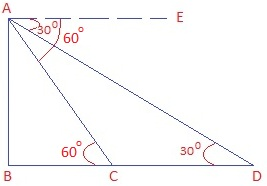# A straight highway leads to the foot of a tower. A man standing at the top of the tower observes a car at an angle of depression of 30°, which is approaching the foot of the tower with a uniform speed. Six seconds later, the angle of depression of the car is found to be 60°. Find the time taken by the car to reach the foot of the tower from this point.

Let AB be the tower. D is the initial and C is the final position of the car respectively.

As man is standing at the top of the tower so, Angles of depression are measured from A.

BC is the distance from the foot of the tower to the car.From ΔABC, tan 60° = AB/BC

√3 = AB/BC

BC = AB/√3

AB = √3 BC

In right ΔABD,

tan 30° = AB/BD

1/√3 = AB/BD

AB = BD/√3

Now,

√3 BC = BD/√3 (Since LHS are same, so RHS are also same)

3 BC = BD

3 BC = BC + CD

2BC = CD or BC = CD/2

Here, distance of BC is half of CD.

Hence, the time taken is also half.

Time taken by car to travel distance CD = 6 sec.

Time taken by car to travel BC = 6/2 = 3 sec.